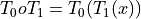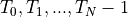# Copy a Non-CompositeTransform#

## Synopsis#

Copy a non-CompositeTransform.

## Results#

```Original transform: Euler3DTransform (0x132e880)
RTTI typeinfo:   itk::Euler3DTransform<float>
Reference Count: 2
Modified Time: 5
Debug: Off
Object Name:
Observers:
none
Matrix:
0.930432 -0.294044 0.218711
0.308577 0.950564 -0.0347626
-0.197677 0.0998334 0.97517
Offset: [3.63622, 5.66636, 5.62082]
Center: [-3.5, -4.5, -5.5]
Translation: [4, 5, 6]
Inverse:
0.930432 0.308577 -0.197677
-0.294044 0.950564 0.0998333
0.218711 -0.0347626 0.97517
Singular: 0
Euler's angles: AngleX=0.1 AngleY=0.2 AngleZ=0.3
m_ComputeZYX = 0

Transform copy: Euler3DTransform (0x132eda0)
RTTI typeinfo:   itk::Euler3DTransform<float>
Reference Count: 3
Modified Time: 10
Debug: Off
Object Name:
Observers:
none
Matrix:
0.930432 -0.294044 0.218711
0.308577 0.950564 -0.0347626
-0.197677 0.0998334 0.97517
Offset: [3.63622, 5.66636, 5.62082]
Center: [-3.5, -4.5, -5.5]
Translation: [4, 5, 6]
Inverse:
0.930432 0.308577 -0.197677
-0.294044 0.950564 0.0998333
0.218711 -0.0347626 0.97517
Singular: 0
Euler's angles: AngleX=0.1 AngleY=0.2 AngleZ=0.3
m_ComputeZYX = 0
```

## Code#

### C++#

```#include "itkEuler3DTransform.h"

int
main()
{
using TransformType = itk::Euler3DTransform<float>;

auto                          transform = TransformType::New();
TransformType::ParametersType parameters(6);
parameters = 0.1;
parameters = 0.2;
parameters = 0.3;
parameters = 4.0;
parameters = 5.0;
parameters = 6.0;
transform->SetParameters(parameters);

TransformType::FixedParametersType fixedParameters(3);
fixedParameters = -3.5;
fixedParameters = -4.5;
fixedParameters = -5.5;
transform->SetFixedParameters(fixedParameters);
std::cout << "Original transform: " << transform << std::endl;

TransformType::Pointer transformCopy = transform->Clone();
std::cout << "Transform copy: " << transformCopy << std::endl;

return EXIT_SUCCESS;
}
```

## Classes demonstrated#

template<typename TParametersValueType, unsigned int NInputDimensions = 3, unsigned int NOutputDimensions = 3>
class Transform : public itk::TransformBaseTemplate<TParametersValueType>

Transform points and vectors from an input space to an output space.

This abstract class defines the generic interface for a geometric transformation from one space to another. The class provides methods for mapping points, vectors and covariant vectors from the input space to the output space.

Given that transformations are not necessarily invertible, this basic class does not provide the methods for back transformation. Back transform methods are implemented in derived classes where appropriate.

Another requirement of the registration framework is the computation of the transform Jacobian. In general, an

ImageToImageMetric requires the knowledge of the Jacobian in order to compute the metric derivatives. The Jacobian is a matrix whose element are the partial derivatives of the output point with respect to the array of parameters that defines the transform.
Registration Framework Support

Typically a Transform class has several methods for setting its parameters. For use in the registration framework, the parameters must also be represented by an array of doubles to allow communication with generic optimizers. The Array of transformation parameters is set using the SetParameters() method.

Subclasses must provide implementations for:

virtual OutputPointType

TransformPoint(const InputPointType &) const

virtual OutputVectorType

TransformVector(const InputVectorType &) const

virtual OutputVnlVectorType

TransformVector(const InputVnlVectorType &) const

virtual OutputCovariantVectorType

TransformCovariantVector(const InputCovariantVectorType &) const

virtual void

SetParameters(const ParametersType &)

virtual void

SetFixedParameters(const FixedParametersType &)

virtual void ComputeJacobianWithRespectToParameters( const InputPointType &, JacobianType &) const

virtual void ComputeJacobianWithRespectToPosition( const InputPointType & x, JacobianPositionType &jacobian ) const;

Since TranformVector and TransformCovariantVector have multiple overloaded methods from the base class, subclasses must specify:

using Superclass::TransformVector;

using Superclass::TransformCovariantVector;

Subclassed by itk::MatrixOffsetTransformBase< TParametersValueType, NInputDimensions, NOutputDimensions >, itk::MatrixOffsetTransformBase< TParametersValueType, 2, 2 >, itk::MatrixOffsetTransformBase< TParametersValueType, 3, 3 >, itk::MatrixOffsetTransformBase< TParametersValueType, NDimensions, NDimensions >

template<typename TParametersValueType = double, unsigned int NDimensions = 3>
class CompositeTransform : public itk::MultiTransform<TParametersValueType, NDimensions, NDimensions>

This class contains a list of transforms and concatenates them by composition.

This class concatenates transforms in reverse queue order by means of composition:Transforms are stored in a container (queue), in the following order:Transforms are added via a single method, AddTransform(). This adds the transforms to the back of the queue. A single method for adding transforms is meant to simplify the interface and prevent errors. One use of the class is to optimize only a subset of included transforms.

The sub transforms are the same dimensionality as this class.

Example: A user wants to optimize two Affine transforms together, then add a Deformation Field (DF) transform, and optimize it separately. He first adds the two Affines, then runs the optimization and both Affines transforms are optimized. Next, he adds the DF transform and calls SetOnlyMostRecentTransformToOptimizeOn, which clears the optimization flags for both of the affine transforms, and leaves the flag set only for the DF transform, since it was the last transform added. Now he runs the optimization and only the DF transform is optimized, but the affines are included in the transformation during the optimization.

Optimization Flags: The m_TransformsToOptimize flags hold one flag for each transform in the queue, designating if each transform is to be used for optimization. Note that all transforms in the queue are applied in TransformPoint, regardless of these flags states’. The methods GetParameters, SetParameters, ComputeJacobianWithRespectToParameters, GetTransformCategory, GetFixedParameters, and SetFixedParameters all query these flags and include only those transforms whose corresponding flag is set. Their input or output is a concatenated array of all transforms set for use in optimization. The goal is to be able to optimize multiple transforms at once, while leaving other transforms fixed. See the above example.

Setting Optimization Flags: A transform’s optimization flag is set when it is added to the queue, and remains set as other transforms are added. The methods SetNthTransformToOptimize* and SetAllTransformToOptimize* are used to set and clear flags arbitrarily. SetOnlyMostRecentTransformToOptimizeOn is a convenience method for setting only the most recently added transform for optimization, with the idea that this will be a common practice.

Indexing: The index values used in GetNthTransform and SetNthTransformToOptimize* and SetAllTransformToOptimize* follow the order in which transforms were added. Thus, the first transform added is at index 0, the next at index 1, etc.

Inverse: The inverse transform is created by retrieving the inverse from each sub transform and adding them to a composite transform in reverse order. The m_TransformsToOptimizeFlags is copied in reverse for the inverse.